Successfully reported this slideshow.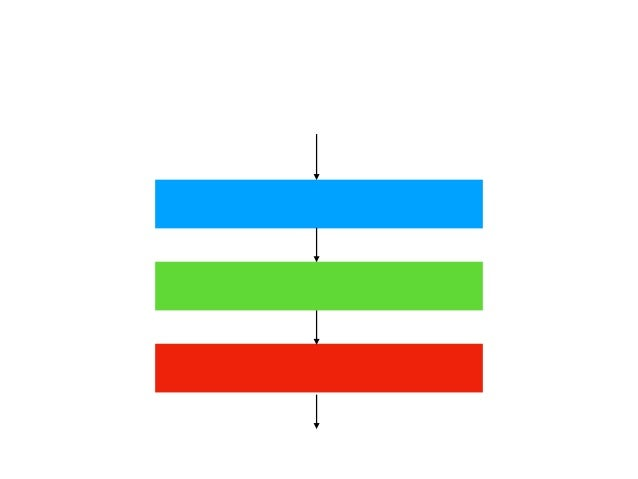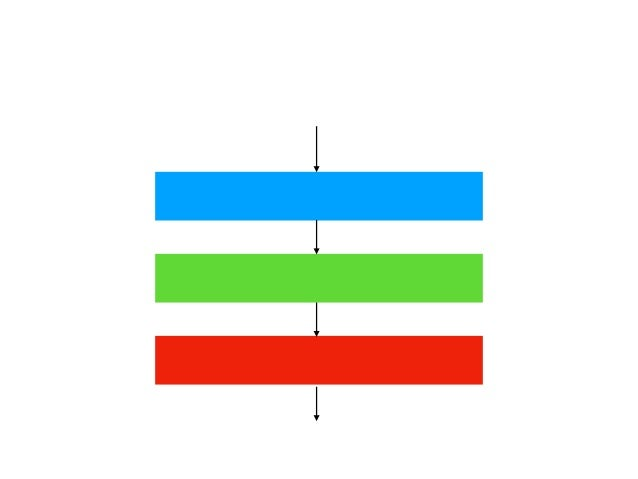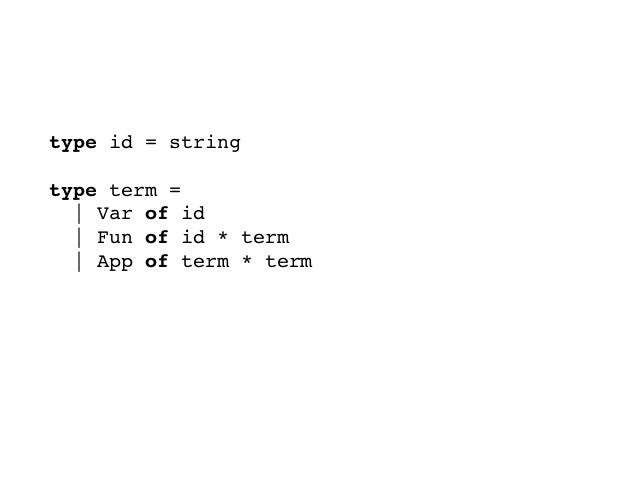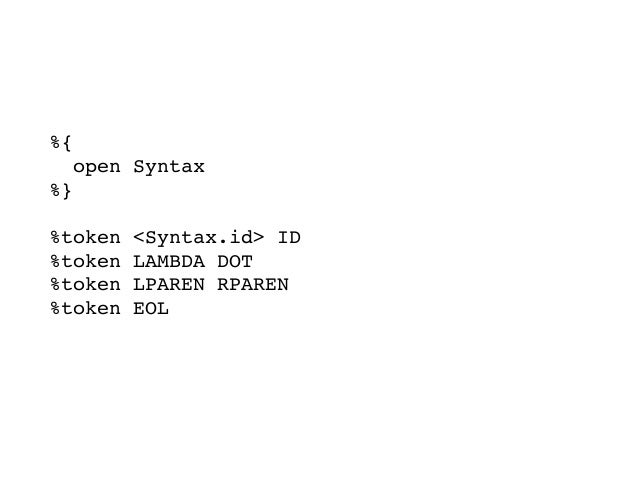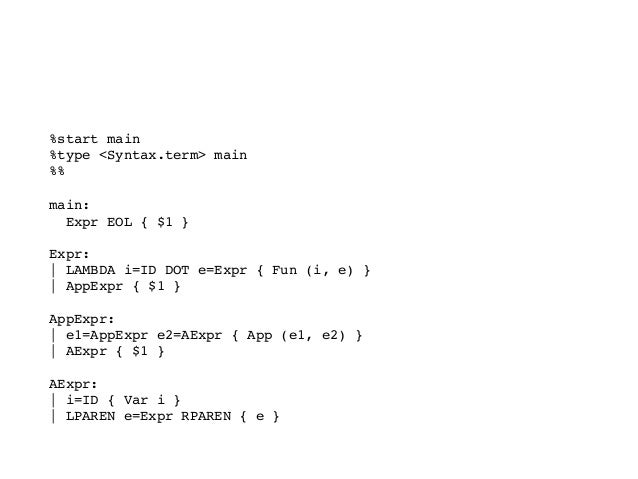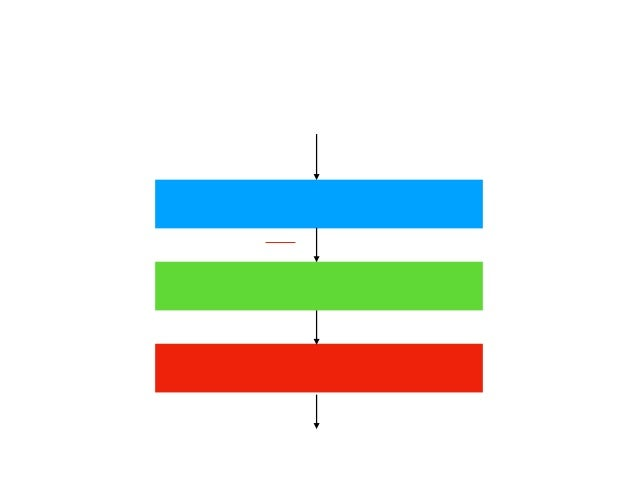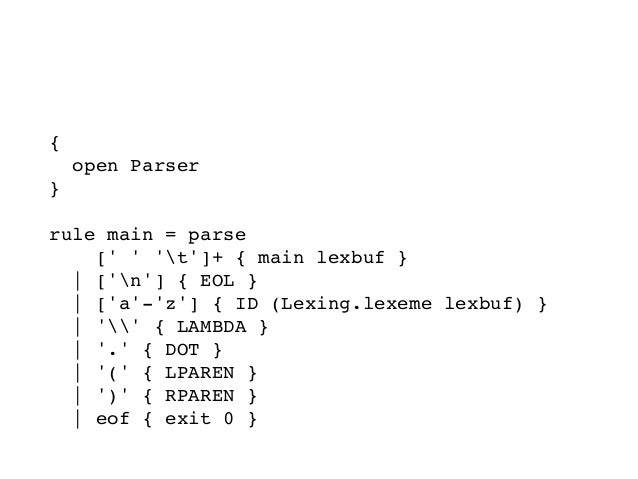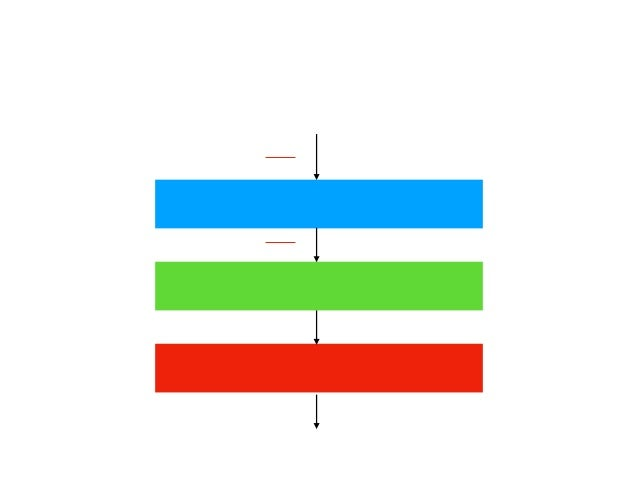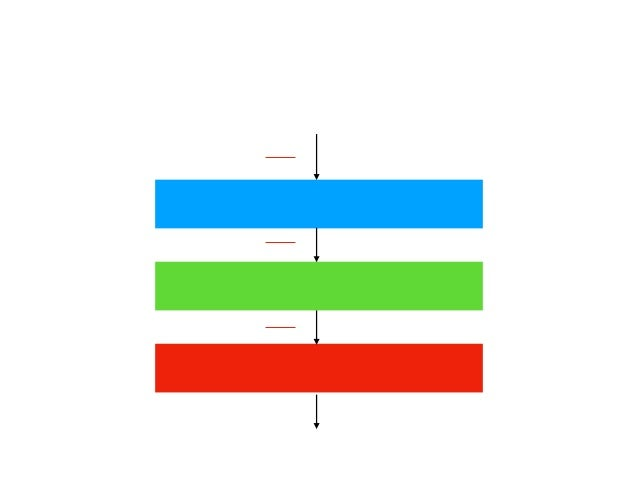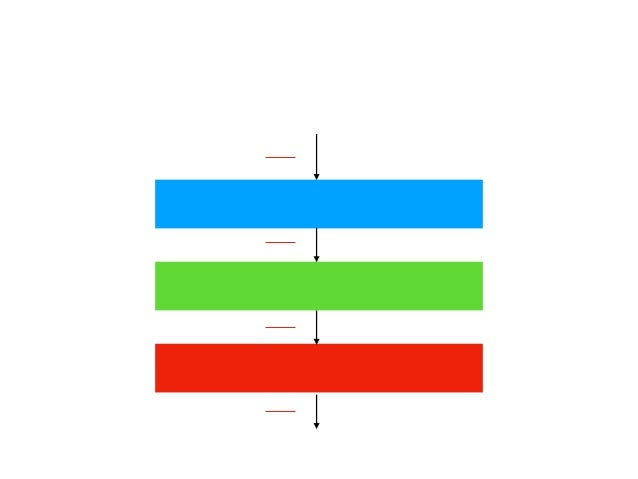Upcoming SlideShare
×

# つくってあそぼ ラムダ計算インタプリタ

2018/10/18 KMC例会講座 型無しラムダ計算のインタプリタを実装してみる

• Full Name
Comment goes here.

Are you sure you want to Yes No• Be the first to comment

### つくってあそぼ ラムダ計算インタプリタ

1. 1. つくってあそぼ ラムダ計算インタプリタ 2018/10/18 KMC例会講座 @utgwkk
2. 2. 自己紹介 • @utgwkk (うたがわきき) • 工学部情報学科4回生 • プログラミング言語に関する研究 • Euro Truck Simulator 2
3. 3. 参考  Urzyczyn et al. Lectures on the Curry-Howard Isomorphism, Studies in Logic and the Foundations of Mathematics, 149, Elsevier Science  http://www.kb.ecei.tohoku.ac.jp/ sumii/class/ keisanki-software-kougaku-2005/lambda.pdf  http://ttic.uchicago.edu/ pl/classes/CMSC336- Winter08/lectures/lec4.pdf
4. 4. おしながき • 動機 • λ計算について知ろう • λ計算のインタプリタを実装しよう
5. 5. 動機 • λ計算の見た目はコンパクトでかつ表現力が強い • しかし実装をしたことがない • 🔜インタプリタを実装してみよう • 論文読むのがだるいときに手を動かしてた
6. 6. この講座の目的 • 「みなさんが普段使っているプログラミング言語(のイン タプリタ)もこのように実装されている」という実感を持っ てもらう • λ計算を実装したときのつまずきポイントを共有する
7. 7. おしながき • 動機 • λ計算について知ろう • λ計算のインタプリタを実装しよう
8. 8. (型無し)λ計算 • 計算のモデル化のひとつ • 関数をつくる(抽象) • 関数を適用する • これだけでなんとチューリング完全
9. 9. λ項 • M ::= x ¦ λx.M ¦ M M • xは変数 • 関数抽象は右結合 λx.λy.M = λx.(λy.M) • 関数適用は左結合 PQR = (PQ)R • 適当に括弧を加えてよい
10. 10. さまざまなλ項 • (λx.x)y • λx.λy.x • λx.λy.λz.xz(yz)
11. 11. 束縛変数と自由変数 • 束縛変数 • 関数抽象によって束縛されている変数 • λx.λy.xyz の束縛変数はx,y • 自由変数 • 束縛変数ではないもの • λx.λy.xyz の自由変数はz
12. 12. 変数の置換 • M[x:=N] • 項Mに登場する自由変数xを • (差し支えない範囲で)項Nに置換する • 差し支えない範囲ってなに？
13. 13. 変数の置換の例 • x[x:=λy.y] = λy.y • y[x:=λz.z] = y (x y) • (x z (y x))[x:=λw.w] = (λw.w) z (y (λw.w)) • λx.x[x:=λy.y] = λx.x • λy.x[x:=λz.z] = λy.λz.z (x y)
14. 14. α変換 • λx.M =α λy.M[x:=y] • 差し支えない範囲で引数の変数名は本質ではない • λx.x と λy.y を区別する必要はないですよね？
15. 15. β簡約 • ざっくり言えば関数適用 • (λx.M) N -> M[x:=N]
16. 16. β簡約を体感する • def f(x): return x + x • f(2) = 2 + 2 (= 4) • // これはあくまで体感です
17. 17. β簡約 (λx.λy.λz.xz(yz)) (λx.λy.x) (λx.λy.x) ->(λy.λz.(λx.λy.x)z(yz)) (λx.λy.x) ->λz.(λx.λy.x)z((λx.λy.x)z) ->λz.(λy.z)((λx.λy.x)z) ->λz.(λy.z)(λy.z) ->λz.z
18. 18. 正規形 • これ以上β簡約できない項 • 例 • x • λx.x • λx.λy.x
19. 19. おしながき • 動機 • λ計算について知ろう • λ計算のインタプリタを実装しよう
20. 20. 仕様 • 入力: λ項の文字列表現 • 出力: β簡約のステップと正規形(のようなもの) • (じつは必ずしも正規形に簡約されるわけではない) • (β簡約してみて，項が変化しなかったら停止する)
21. 21. 実装言語 • 言語はOCamlを使う • そこそこツールチェーンがある • わたしがよく触っている • 使いこなせてはいないと思う……
22. 22. 簡約までの流れ トークン列 (LAMBDA ID DOT ...) 構文木 (Fun (x, ...)) De Bruijn index (Fun ...) (λx.λy.λz.xz(yz))(λx.λy.x)(λx.λy.x) λx.x 字句解析 構文解析 De Bruijn indexへ変換 β簡約
23. 23. 実装の流れ トークン列 (LAMBDA ID DOT ...) 構文木 (Fun (x, ...)) De Bruijn index (Fun ...) (λx.λy.λz.xz(yz))(λx.λy.x)(λx.λy.x) λx.x 字句解析 構文解析 De Bruijn indexへ変換 β簡約 (1) (2) (3) (4)
24. 24. cf. インタプリタ トークン列 (ID(x) EQ NUM(1) ...) 構文木 (STMT([ASSIGN("x", ...), ...]) 仮想機械コード (LOAD 1, STORE 0, ...) x = 1; x + 1 2 字句解析 構文解析 仮想機械コードへの変換 評価 ※どれくらいデータ構造を変換するかは設計による
25. 25. λ項のデータ型を定義しよう • M ::= x ¦ λx.M ¦ M M • 忠実に落としこむ
26. 26. λ項のデータ型を定義しよう type id = string    type term =  | Var of id  | Fun of id * term  | App of term * term
27. 27. 実装の流れ トークン列 (LAMBDA ID DOT ...) 構文木 (Fun (x, ...)) De Bruijn index (Fun ...) (λx.λy.λz.xz(yz))(λx.λy.x)(λx.λy.x) λx.x 字句解析 構文解析 De Bruijn indexへ変換 β簡約 (1) (2) (3) (4)
28. 28. 構文解析 • λx.x のような記号列に意味を持たせる作業 • λx.x -> Fun (x, Var x) • (厳密にはこの記号列をそのまま扱うわけではない)
29. 29. 構文解析器を実装しよう • menhirを使うよ • yaccのOCaml版のすごいやつ • ocamlyaccというのもある • 文法をBNF風のもので記述
30. 30. トークンを定義する • M ::= x ¦ λx.M ¦ M M • グッと睨んで必要そうなトークンを定義しよう • ID (変数) • LAMBDA, DOT (λ, .) • LPAREN, RPAREN (括弧) • EOL (λ項の終端)
31. 31. トークンを定義する %{  open Syntax  %}    %token <Syntax.id> ID  %token LAMBDA DOT  %token LPAREN RPAREN  %token EOL
32. 32. 文法を定義する • M ::= x ¦ λx.M ¦ M M • グッと睨んで文法を書く • <main> ::= <Expr> EOL • <Expr> ::= LAMBDA ID DOT <Expr> ¦ <AppExpr> • <AppExpr> ::= <AppExpr> <AExpr> ¦ <AExpr> • <AExpr> ::= ID ¦ LPAREN <Expr> RPAREN
33. 33. 文法を定義する %start main  %type <Syntax.term> main  %% main:  Expr EOL { \$1 }    Expr:  | LAMBDA i=ID DOT e=Expr { Fun (i, e) }  | AppExpr { \$1 }    AppExpr:  | e1=AppExpr e2=AExpr { App (e1, e2) }  | AExpr { \$1 }    AExpr:  | i=ID { Var i }  | LPAREN e=Expr RPAREN { e }
34. 34. 実装の流れ トークン列 (LAMBDA ID DOT ...) 構文木 (Fun (x, ...)) De Bruijn index (Fun ...) (λx.λy.λz.xz(yz))(λx.λy.x)(λx.λy.x) λx.x 字句解析 構文解析 De Bruijn indexへ変換 β簡約 (1) (2) (3) (4)
35. 35. 字句解析 • そもそもλx.xのような文字列のままだと扱いづらい • 空白文字を無視するとか • コメントもここで取り除かれる • LAMBDA ID(x) DOT ID(x) ぐらいに明確な列だとよい
36. 36. 字句解析器を実装しよう • ocamllexを使うよ • lexのOCaml版 • ルールを正規表現で記述
37. 37. 字句解析ルールを定義する {  open Parser  }    rule main = parse  [' ' 't']+ { main lexbuf }  | ['n'] { EOL }  | ['a'-'z'] { ID (Lexing.lexeme lexbuf) }  | '' { LAMBDA }  | '.' { DOT }  | '(' { LPAREN }  | ')' { RPAREN }  | eof { exit 0 }
38. 38. 実装の流れ トークン列 (LAMBDA ID DOT ...) 構文木 (Fun (x, ...)) De Bruijn index (Fun ...) (λx.λy.λz.xz(yz))(λx.λy.x)(λx.λy.x) λx.x 字句解析 構文解析 De Bruijn indexへ変換 β簡約 (1) (2) (3) (4)
39. 39. De Bruijn indexに変換しよう • ……ってなに？
40. 40. α変換(思い出す) • 「束縛変数の名前は本質ではない」 • いっぽう構文木には束縛変数の名前がある • 🔜適切に変数の名前のことを考えないと  簡約結果がめちゃくちゃになる • 🔜束縛変数の名前に束縛されたくない！！！！
41. 41. β簡約(まちがい) (λx.λy.λz.xz(yz)) (λx.λz.x) (λx.λy.x) ->(λy.λz.(λx.λz.x)z(yz)) (λx.λy.x) ->λz.(λx.λz.x)z((λx.λy.x)z) ->λz.(λz.z)((λx.λy.x)z) ->λz.(λz.z)(λy.z) ->λz.λy.z
42. 42. De Bruijn indexに変換しよう • 関数抽象による束縛からのネストの深さで変数が一意に 定まる • ここでは0-indexとする • λx.λy.x -> λ.λ.1 • λx.λy.λz.xz(yz) -> λ.λ.λ.2 0 (1 0) • ただし自由変数は放っておく
43. 43. De Bruijn indexの強み • 変数の名前を構文木から取り除くことができる • 簡約の過程でのα変換を考えなくてよい
44. 44. De Bruijn indexへの変換 • D(Γ, N, x) = N - Γ(x) (Γ(x)が定義されている) • D(Γ, N, x) = x (Γ(x)が定義されない，自由変数である) • D(Γ, N, λx.M) = λ.(D(Γ{x:N+1}, N+1, M)) • D(Γ, N, PQ) = (D(Γ, N, P))(D(Γ, N, Q)) • Dmain(M) = D({}, 0, M)
45. 45. De Bruijn indexへの変換 • 例 • Dmain(λx.λy.x) • = D({},0,λx.λy.x) • = λ.(D({x:1},1,λy.x)) • = λ.λ.(D({x:1,y:2},2,x)) • = λ.λ.(2-1) = λ.λ.1
46. 46. 実装の流れ トークン列 (LAMBDA ID DOT ...) 構文木 (Fun (x, ...)) De Bruijn index (Fun ...) (λx.λy.λz.xz(yz))(λx.λy.x)(λx.λy.x) λx.x 字句解析 構文解析 De Bruijn indexへ変換 β簡約 (1) (2) (3) (4)
47. 47. De Bruijn indexのβ簡約 • 機械的なindexの操作と置換だけでβ簡約が実行される • (なんかすごい式) • 資料を参照
48. 48. 実装の流れ トークン列 (LAMBDA ID DOT ...) 構文木 (Fun (x, ...)) De Bruijn index (Fun ...) (λx.λy.λz.xz(yz))(λx.λy.x)(λx.λy.x) λx.x 字句解析 構文解析 De Bruijn indexへ変換 β簡約 (1) (2) (3) (4)
49. 49. 実装したもの • https://github.com/utgwkk/lambda-chama
50. 50. デモ • (x.y.z.xz(yz))(x.y.x)(x.y.x) • (x.y.z.xz(yz))(x.z.x)(x.y.x) (変数名を変えただけ) • f.(x.f(xx))(x.f(xx)) (停止しない)
51. 51. 今後の課題 • 型を付ける • 実装はしたが講座では省略 • 自由変数の扱いをうまくやりたい
52. 52. まとめ • λ計算についてなんとなく分かってきた • λ計算のインタプリタを実装した